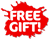ISBN: 978-1-990015-13-7

## Level 2 Mathematics and Statistics Externals Learning WorkbookPhyl Haydock

• 2595

Level 2 Mathematics and Statistics Externals Learning Workbook covers the three externally assessed NCEA Level 2 Mathematics and Statistics Achievement Standards:

• AS91261 Apply algebraic methods in solving problems
• AS91262 Apply calculus methods in solving problems
• AS91267 Apply probability methods in solving problems

These standards are worth a total of 13 credits.

The workbook features:

• bite-sized sections of notes with student-friendly explanations
• worked examples to illustrate fundamental concepts
• plenty of exercises so students can improve their skills and practise problem solving
• answers to all exercises (provided at the end of the book)

An index gives easy access to important terms and definitions highlighted in the text.

This workbook provides a variety of questions for the student. Basic problems require fundamental concepts to be applied in straightforward algebra, calculus or probability contexts. More challenging applications require strategies to be devised and clearly communicated, in order to solve harder theoretical or practical problems.

The concise notes and write-on exercises keep student work together in a compact format, providing a useful end-of-topic revision tool.

This workbook is ideal for use in the classroom or for homework, home study and revision.

Published: 11/2020